# Civil Engineering - Theory of Structures - Discussion

### Discussion :: Theory of Structures - Section 1 (Q.No.21)

21.

In case of a simply supported rectangular beam of span L and loaded with a central load W, the length of elasto-plastic zone of the plastic hinge, is

 [A].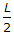[B].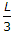[C].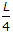[D].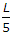[E].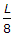Explanation:

No answer description available for this question.

 Anshul Gupta said: (Sep 25, 2013) Shape factor = 1.5. Plastic moment/elastic moment = 1.5. 1.5 = L/(2x). x = L/3.

 Arghya said: (Dec 23, 2014) I have a problem in that why we consider the elastic moment as 2x?

 Sagnik said: (Feb 28, 2015) It is 1.5 = Mp/Me. 1.5 = (L/2)/X. As plastic hinge will form at L/2.

 Nitu said: (Sep 11, 2015) Length of plastic hinge = Distance between Mp and My. You can use a formula too = L (1-(1/shape factor)). Shape factor for rectangular = 1.5. Plastic length = L/3.

 Zubair Ahmad said: (Mar 8, 2016) S.F = Plastic Moment/Elastic moment. 1.5(for rect. section) = L/2/x (plastic hinge at (L/2). 1.5 = L/2x. x = L/1.5X2. x = L/3.

 Rana Muhammad Ashraf said: (Aug 12, 2016) X is what distance? can anyone explain.

 Chandan said: (Sep 11, 2016) X is the distance from one end to plastic hinge.

 Steve said: (Jul 31, 2018) I think x is any distance along the beam where strain can occur under a considerable amount of stress. hence, the modulus of elasticity is applicable here. Also, L/2 is where ultimate failure is likely to occur and hence it is where the plastic hinge is located, hence, the plastic modulus is applicable here and that is the idea of having the ratio as 0.5L/X=1.5. Therefore X=0.33333333L=L/3.

 Nungshi said: (Aug 30, 2018) Lp/L =(1-1/Ks) where Ks is shape factor. (For centrally concentrated load). Lp/L = √(1-1/Ks) (for udl).

 Dhananjay said: (Feb 23, 2019) Thanks for explaining @Nitu.

 Opsc Aee said: (Nov 21, 2019) For I-section - L/8. Rectangular section - L/3.

 Vignesh said: (Feb 16, 2020) Can we call the ratio between plastic moment to elastic moment as the ELASTOPLASTIC zone?

 Kaushik said: (Jul 20, 2020) For I- section it is (L/5).